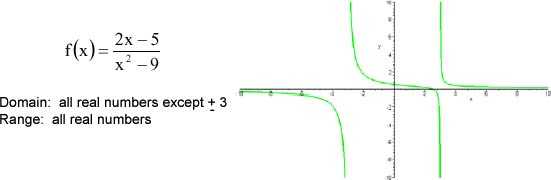Graphing Relations, Domain, and Range

Graphing Relations, Domain, and Range

relation is just a relationship between sets of information.  When x and y values are linked in an equation or inequality, they are related; hence, they represent a relation.

Not all relations are functions.  A function states that given an x, we get one and only one y

 y = 3x + 1 x2 + y2 = 5 This is a function.  For any value of x you plug in, you will get only one possible value for y.  For example, if x = 2, y can only equal 7. This is not a function.  Any value of x can give you more than one possible y.  For example, if x = 1, y could equal 2 or −2.

It is possible to test a graph to see if it represents a function by using the vertical line test.  Given the graph of a relation, if you can draw a vertical line that crosses the graph in more than one place, then the relation is not a function.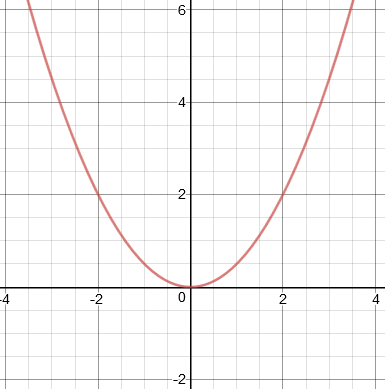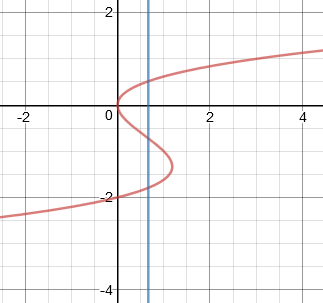This is a function.  Any vertical line will cross this graph at only one point. This is not a function.There is a vertical line that will cross this graph at more than one point.

The domain is defined as all the possible input values (usually x) which allow the formula to work.  Note that values that cause a denominator to be zero, which makes the function undefined, are not allowable values.

• The function y = 4x2 − 9 has a domain of all real numbers, which can be expressed using the interval (−∞, ∞).  Every possible x-value will give you a legitimate y-value.
• The function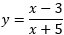has a domain of all real numbers except −5, because when x = −5, the denominator will be zero, and the function will be undefined.  We can express this using the inequality x ≠ −5, or the interval (−∞, −5) ⋃ (−5, ∞).

The range is the set of all possible output values (usually y), which result from using the formula.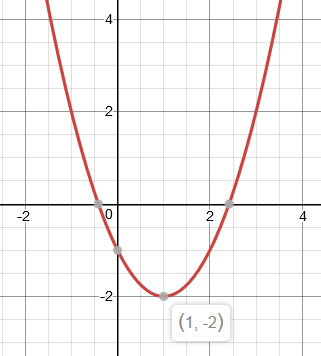If you graph the function y = x2 − 2x − 1, you'll see that the y-values begin at −2 and increase forever.  The range of this function is all real numbers from −2 onward.  We can express this using the interval [−2, ∞).

Practice

For each relation, state if it is a function.  State the domain and range, and sketch a graph.

1.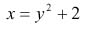2.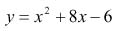3.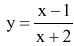4.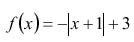5.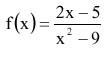1. Not a function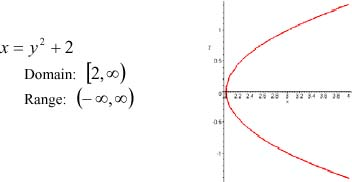2.  Function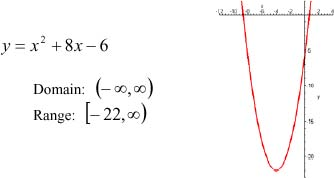3. Function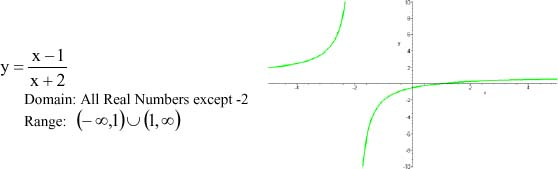4. Function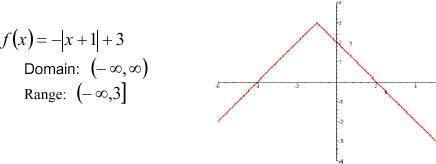5. Function